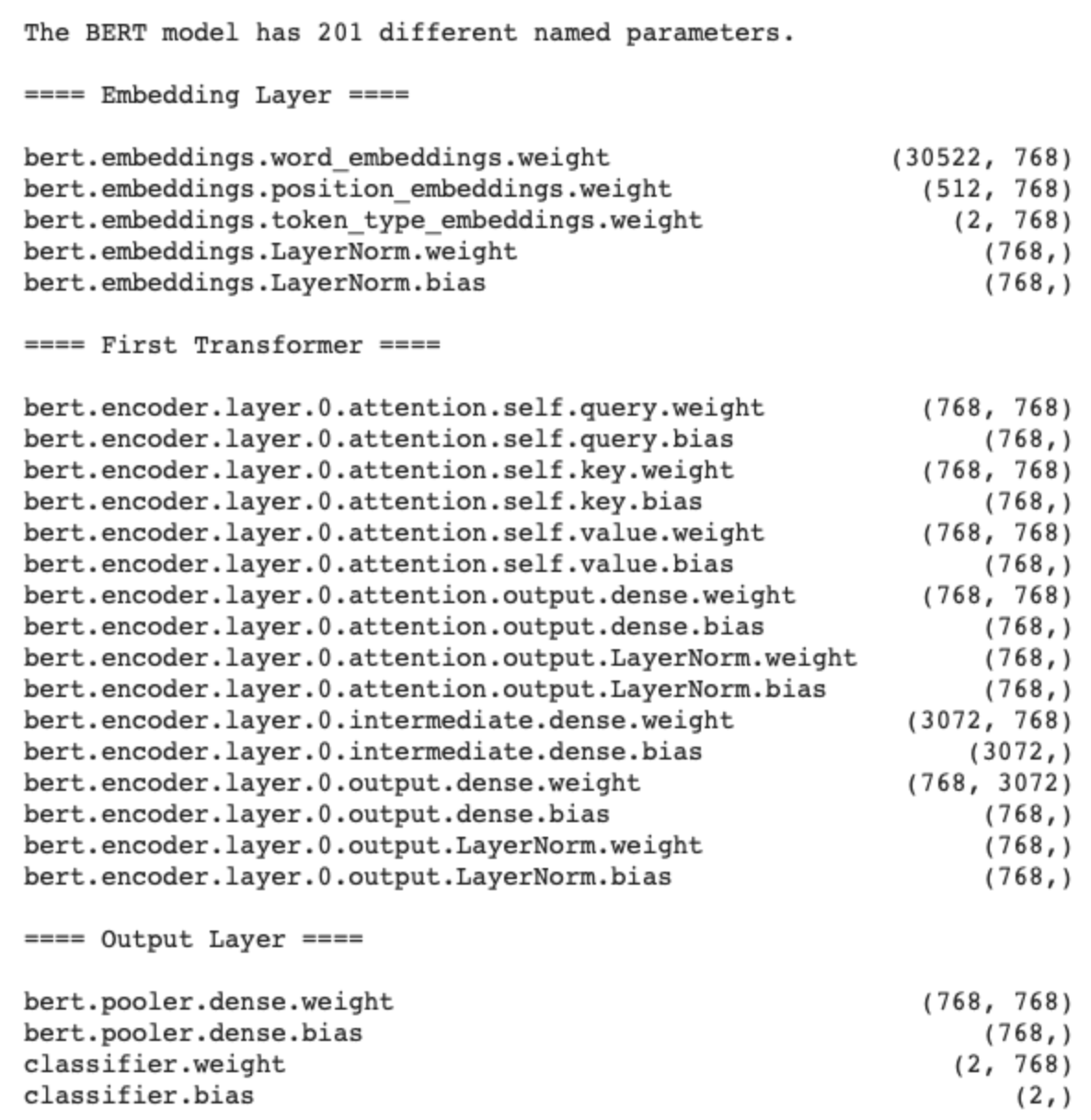# Step-by-Step BERT Explanation & Implementation Part 3 — Training & Testing

Original article was published by Phillip Kim on Deep Learning on Medium# Step-by-Step BERT Explanation & Implementation Part 3 — Training & Testing

This is Part 3 of the BERT Explanation & Implementation series. If you have not read Part 2 yet, it’s best to pick up from there. Let us continue from where we left off.

`param_optimizer = list(model.named_parameters())no_decay = ['bias', 'gamma', 'beta']optimizer_grouped_parameters = [{'params': [p for n, p in param_optimizer if not any(nd in n for nd in no_decay)], 'weight_decay_rate': 0.01}, {'params': [p for n, p in param_optimizer if any(nd in n for nd in no_decay)], 'weight_decay_rate': 0.0}]`

We get weights for various layers and put them into a single list. Once that is done, we separate weight parameters from bias, gamma, and beta parameters. We filter one group without these values and another with them. Hence you see one group with weight_decay_rate 0.01 and another 0.0.

`params = param_optimizer.copy()print('The BERT model has {:} different named parameters.\n'.format(len(params)))print('==== Embedding Layer ====\n')for p in params[0:5]:    print("{:<55} {:>12}".format(p, str(tuple(p.size()))))print('\n==== First Transformer ====\n')for p in params[5:21]:    print("{:<55} {:>12}".format(p, str(tuple(p.size()))))print('\n==== Output Layer ====\n')for p in params[-4:]:    print("{:<55} {:>12}".format(p, str(tuple(p.size()))))`

You can check the number of parameters that BERT has using the code above.

`optimizer = AdamW(optimizer_grouped_parameters,lr=2e-5)scheduler = get_linear_schedule_with_warmup(optimizer, num_warmup_steps=100, num_training_steps=1000)`

We set up optimizer and scheduler needed for learning. We use default values for them.

`def flat_accuracy(preds, labels):    pred_flat = np.argmax(preds, axis=1).flatten()    labels_flat = labels.flatten()    return np.sum(pred_flat == labels_flat) / len(labels_flat)`

We define a function that calculates accuracy.

`train_loss_set = []epochs = 4for _ in trange(epochs, desc="Epoch"):      model.train()    tr_loss = 0    nb_tr_examples, nb_tr_steps = 0, 0    for step, batch in enumerate(train_dataloader):        batch = tuple(t.to(device) for t in batch)        b_input_ids, b_input_mask, b_labels = batch        optimizer.zero_grad()        loss = model(b_input_ids, token_type_ids=None, attention_mask=b_input_mask, labels=b_labels)        loss = loss        train_loss_set.append(loss.item())            loss.backward()        optimizer.step()        scheduler.step()        tr_loss += loss.item()        nb_tr_examples += b_input_ids.size(0)        nb_tr_steps += 1print("Train loss: {}".format(tr_loss/nb_tr_steps))save_dict(category_to_id, id_to_category)tokenizer.save_pretrained('bert_model/')model.save_pretrained("bert_model/")`

We use 4 epochs, and for each epoch, we set optimizer to be initialized with .zero_grad() as the gradients accumulate by default in pytorch. We perform a forward pass (input values into the model). We accumulate the training loss over all of the batches so we can calculate average loss at the end. Then, we do backward pass to get gradients. Loss is a single tensor value and loss.item() returns a python value for the loss. We, then, save the results.

`model.eval()eval_loss, eval_accuracy = 0, 0nb_eval_steps, nb_eval_examples = 0, 0    for batch in validation_dataloader:        batch = tuple(t.to(device) for t in batch)        b_input_ids, b_input_mask, b_labels = batch        with torch.no_grad():            (loss, logits) = model(b_input_ids, token_type_ids=None, attention_mask=b_input_mask, labels=b_labels)        logits = logits.cpu().numpy()        label_ids = b_labels.to('cpu').numpy()        tmp_eval_accuracy = flat_accuracy(logits, label_ids)            eval_accuracy += tmp_eval_accuracy        nb_eval_steps += 1print("Validation Accuracy: {}".format(eval_accuracy/nb_eval_steps))`

We set the model to evaluation mode. We only do forward pass but no backward pass as we are not training a model at this point. Logits are the output values prior to applying an activation function like softmax function. We move logits from GPU to CPU and calculate evaluation accuracy.

Our evaluation results show very high accuracy. This chapter ends the series but I will post more on how to solve other variational NLP problems using BERT models in the near future.